### Temperature and Heat

1. How much heat should be added to boil 500 ml of water that has been sitting at 15 °C? The specific heat capacity of water is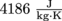.

2. How much energy does it take to heat up a 250 ml cup of coffee from 25 °C to 40 °C? We can assume that the specific heat capacity of coffee is the same as water,.

3. We boil 500 ml of water and get distracted by a Sopranos marathon. When we return to the pot of boiling water, we see the last drop evaporate. The latent heat of vaporization of water is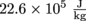. How much energy got wasted by turning all of the pasta water into steam?

4. It takes 200,000 J to cool a glass of milk from boiling down to 0 °C. We will assume that the specific heat capacity of milk is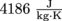. How much milk is in the glass?

5. Webster treated himself to an ice cream float, but he got distracted while counting the birds nearby. The ice cream melted until the ice cream and the root beer achieved the same temperature. In other words: a tragic phase change occurred. There is still a bit of ice cream left floating in Webster's glass, though.

He started out with 100 g of ice cream at 4 °C and 200 g of root beer at 25 °C. The latent heat fusion of ice cream is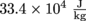and the specific heat capacity of soda is the same as water,. How much ice cream is left when the soda and ice cream temperature have reached equilibrium?

### Thermal Expansion

1. A banister has three metal rods. Rod 1, the handrail, is 5 m long and has a coefficient of linear expansion of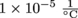. Rod 2, holding up one end, is 2.5 m long and is made of the same material as Rod 1. Lastly, Rod 3, on the other end, is also 2.5 m long and has a coefficient of linear expansion of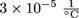. The banister is out in the sun, and on a hot day their temperature increases by 10 °C. Which rod will be the longest rod?

2. Clifford wants to win a bet. He has two 5 m metal rods placed 0.25 m apart from each other lengthwise. The metal rods have coefficients of linear expansion of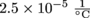. He's thinking about making a bet that without moving these two rods, he could get them to touch each other. How much would the temperature have to rise for these rods to expand until they touch? Assume that the rods will increase in length equally on both ends.

3. Let's try to make Clifford's bet work. We know that different materials have different coefficients of linear expansion. Maybe there's a material with a coefficient of linear expansion large enough that he could win. What coefficient of linear expansion is needed to grow each 5 m rod by 0.25 m, if we are raising the temperature of the two rods by 100 °C?

4. Gus and Gina have an empty aluminum gas tank in their backyard, and they want to fill it up. Where they live, temperatures can fluctuate up to 40 °C over the course of a day. The tank is a cubic tank of height 0.5 m. Gasoline has a coefficient of volumetric expansion of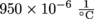, and aluminum has a coefficient of volumetric expansion of 69 × 10-6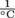. If they fill the tank to the very top with gasoline, would it flow over on a day with a 40 °C change in temperature?

5. Danny, the self-styled fix-it-himself guru, decides to take on yet another DIY home improvement project. Let's all sigh in unison. He is going to pour concrete for a new driveway. He pours concrete for a 50 m2 area that is completely surrounded on three sides by the house and the garage. He leaves no gap between the driveway and the surrounding buildings. Given that concrete has a coefficient of linear expansion of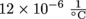and temperatures can increase by 40 °C in a single day, how much could his concrete pad expand in square centimeters?

### The Ideal Gas Law

1. We compress a balloon while maintaining a constant temperature. It started out with a volume of 0.15 m3, and the pressure in the balloon started at 1.01 × 105 Pa. By compressing the balloon, the pressure increases to 2.02 × 105 Pa. How much does the volume of the balloon change?

2. At a temperature of 315 K, a gas has a volume of 0.2 m3 and a pressure of 2 × 105 Pa. How many moles of this gas are present?

3. How many molecules of gas are present in question 2?

4. Little Ella has roped her big brother into participating in her magic show. Since he just read the Shmoop chapter on the ideal gas law, he decides to pop a balloon by holding it above a heating plate. It's not magic if the balloon is directly on the plate—even Ella's 1st grade friends won't be impressed by that. If the temperature 2 feet above the heating plate is 350 K, the balloon has 10 × 1014 molecules of oxygen inside, and the inflated round balloon has a diameter of 12 cm, how much pressure is necessary to put on the balloon as big brother holds it over the heating plate to pop it?

5. Hera compresses a balloon, increasing the pressure on the air in the balloon three-fold and reducing its volume two-fold. By how much does the temperature of the gas change?

### Kinetic Theory of Gases

1. What is the total kinetic energy of a gas containing Avogadro's number of molecules at a temperature of 340 K?

2. What is the average kinetic energy of the gas molecules in question 1?

3. If the mass of each gas molecule from Problems 1 and 2 is 5.36 times; 10-26 kg, what is the average velocity of the gas molecules?

4. The gas from questions 1-3 is in a cubic box with a height of 2 cm. How much force does the gas exert on the box walls?

5. What is the average kinetic energy of a gas molecule at 25 °C? ?

### The First Law of Thermodynamics

1. Draw PV graphs for an (a) isobaric, (b) isothermal, (c), isovolumetic, and (d) adiabatic expansion of an ideal gas and indicate on the graph the amount of work that is done.

2. How much work is done in the process on the PV diagram below?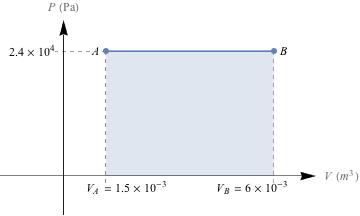3. According to the diagram below, an ideal gas expands either by path A to C, or in a two-step process, A to B followed by B to C. In which process is more work done, and is the work done on the system or by the system(i.e., is the work positive or negative)?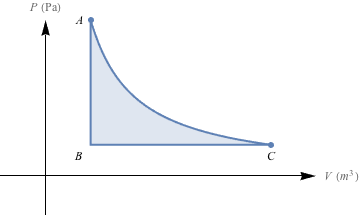4. Fill in the table below, marking whether the quantity is positive, negative, or stays the same for each type of process.

 W Q ΔU Adiabatic - Isothermal + Isovolumetric - Isobaric + +

5. Which of these two thermodynamic cycles experiences the greatest change in internal energy? During which process is more work done?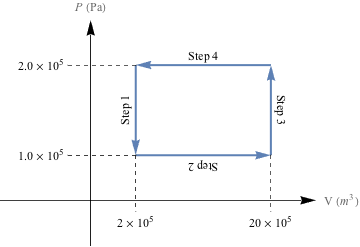Cycle 1 (above)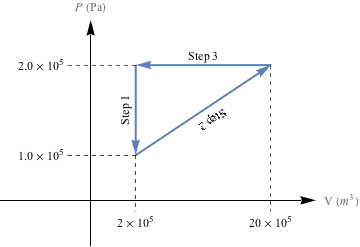Cycle 2 (above)

### The Second Law of Thermodynamics and Heat Engines

1. Max wants to report the efficiency of his heat engine to his physics teacher. It does 300 J of work per cycle, draws 500 J from the warm reservoir and discards 200 J into the cold reservoir. What's the engine's efficiency?

2. Someone asks us to build a heat engine that does 500 J of work per cycle while drawing 300 J from the warm reservoir and discarding 200 J into the cold reservoir. Why is it impossible to build this engine?

3. A heat engine is 60% efficient and draws 800 J of energy from its hot reservoir. How much energy is discarded into the engine's cold reservoir?

4. A heat engine draws 200 J from the hot reservoir and discards 20 J into the cold reservoir. What is this engine's efficiency?

5. The efficiency of a heat engine is 85%, and 50 J are discarded into the engine's cold reservoir. How much work is done by this system?

### The Carnot Cycle

1. A heat engine's cold reservoir has a temperature of 200 K and a hot reservoir at 400 K. What is this engine's Carnot efficiency?

2. If an engine has a heat reservoir at 300 °C and a cold reservoir at 4 °C, can this engine be 80% efficient?

3. An engine runs at a Carnot efficiency of 80%. Its hot reservoir is at a temperature of 600 K. What is the temperature of the cold reservoir?

4. We want to improve (unrealistically) a heat engine's Carnot efficiency from 50% to 90%. The heat reservoir of the engine is 700 K. How much colder should the cold reservoir be to achieve the desired increase in efficiency?

5. What is the increase in Carnot efficiency when a heat engine with a heat reservoir at 500 K and a cold reservoir at 200 K gets a new cold reservoir that can be cooled to 150 K?

### Temperature and Heat Solutions

1. 177,905 Joules

To answer this question, we use the formula Q = cmΔT. The boiling point of water is 100 °C, so ΔT = 100 °C – 15 °C = 85 °C. Celsius is fine for a change in temperature, because the size of each Celsius degrees is equal to a degree in Kelvin. So a change of 85 degrees in Celsius is the same as a change of 85 degrees in Kelvin. 500 ml of water weighs 500 g. Mind the units: those 500 g have to be converted to 0.5 kg to plug it into the equation.

To bring 500 ml of water at 15 °C to a boil we would have to add: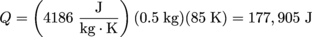Is that a lot? 1 J = 0.239 calories, so we would have to add 42.519 Cal (kilocalories) of energy. The average cup of coffee (brewed, espresso) has about 2.4 Cal, so it takes about 18 cups of coffee to bring a very small pot (500 ml) of water to a boil. That explains why the water is so jittery when it's boiling.

2. 15,698 Joules

We have to convert the 250 ml back to kg again, using 250 ml = 250 g = 0.25 kg. We want to heat the cup of coffee by 15 K (same as Celcius), so: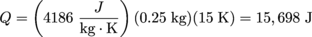3. 11.3 × 105 Joules

When objects undergo phase changes, all of the added energy goes into the phase change and no change in temperature is observed. To calculate the energy that needs to be supplied to change the phase of an object of mass, m (in grams), we use Q = mL, where L is the latent heat of that object. So the amount of heat wasted when 500 ml of water evaporates is: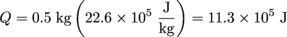4. 478 grams of milk.

As long as we pick the right equation, we can just plug and chug away. We know how much energy we're dealing with, the milk's specific heat capacity, and how big of a temperature change it went through; what we want to know, though, is how much cow juice we're dealing with.

Normally we'd try to find the volume of milk, but using the conversion 1 mL = 1 g, we realize that we can find the mass instead. Good thing, too, since the equation Q = cmΔT contains all of our knowns and unknowns.Converting back to grams, our standard unit of mass, we have 478 g of milk.

5. 0.047 kg of ice cream is all that's left in Webster's glass. To answer this question, we have to think about the special case of phase changes.

During a phase change, all of the heat added to the system goes into changing the phase of the system. The ice cream doesn't increase in temperature while it is melting; it stays at 4 °C. For the ice cream, the equation Q = mL applies.

The root beer, though, does change temperature—heat energy is transferred from the root beer to melt the ice cream, until they are both at the same temperature, 4 °C. Therefore, we also need to use the equation Q = mcΔT. Since all of the heat transferred from the root beer goes into melting the ice cream we equate Qice = Qroot beer, which is mL = mcΔT. Solving time!This 0.053 kg is the amount of ice cream that has melted. To calculate how much ice cream is left in Webster's glass, subtract this value from the original amount of ice cream (in kg) as follows: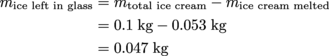### Thermal Expansion Solutions

1. Rod 1 will still be the longest rod.

We use the equation for linear expansion to calculate the change in length for each rod. We're wondering, though, why didn't the builder use the same material for all three parts. Maybe the first two rods are made out of gold, and the budget ran out?

Anyway, the length of Rod 1 is determined by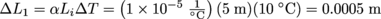. This means Rod 1 will expand by 0.0005 m, so it will be 5.0005 m long.

Next, we calculate the length of Rod 2. Since Rod 2 is made of the same material as Rod 1, its coefficient of linear expansion is also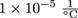.Rod 2 will expand by 0.00025 m, so it will be 2.50025 m long.

Lastly, we approach Rod 3. Same equation, different numbers.Rod 3 will expand by 0.00075 m, so it will be 2.50075 m long in all. Mirror, mirror, on the wall, Rod 1 will be the longest of them all.

2. ΔT = 2000 °C

Clifford knows how to pick an interesting bet, we can say that at least. Since both rods will be increasing in length at the same rate, and with the same coefficient of linear expansion, the rods only have to grow 0.125 m each towards each other to span a gap of 0.25 m.

On top of that, the rods will expand in both directions. To grow 0.125 m towards each other, each rod has to increase in length by 0.25 m total. To determine the change in temperature that would result in an increase in length of 0.25 m of each rod, we use the linear expansion equation.Yikes! Don't make that bet.

3.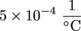We're still using the linear expansion formula, ΔL = αLiΔT, but now we need to solve for α.It might not seem like it to you, but this is still an extremely high coefficient of linear expansion. The bet's still off.

4. Yes, it would.

We have to compare how much the gasoline and the gas tank expand over the course of a blistering-hot day. The volume of the cubic gasoline tank is (0.5 m)3 = 0.125 m3. Completely filling up this tank with gasoline puts the volume of gasoline at 0.125 m3 as well.

Now to see how much everything expands due to the heat. For the tank itself, the change in volume is the following:Meanwhile, the change in volume of the gasoline is:The gasoline increases in volume more than tenfold in comparison the gasoline tank's own volume. Please, Gus and Gina, do not completely fill your tank. A little bit of spilled gas would be the least of your worries. If you screwed on the lid too tight, then the pressure or—gulp—temperature of the gasoline could increase. Not good.

5. 4800 cm2

Let's start by calculating how much Danny's concrete pad will increase in area when the temperature increases by 40 °C. We are given the coefficient of linear expansion, so the coefficient of area expansion is double that,The concrete pad will increase in area by 4800 cm2. How much do the structures surrounding it expand, we wonder? We sense cracks in the making.

### The Ideal Gas Law Solutions

1. It halves.

According to Boyle's law, at a constant temperature we have PV = constant, so P1V1 = P2V2. This allows us to solve for V2, the volume of the balloon after it has been compressed:By doubling the pressure on the balloon we decreased its volume by a factor of 2. Side note: we don't even need the pressure in Pascals: knowing that the second pressure is double the first is enough.

2. 15.28 moles

The ideal gas law, PV = nRT, is just the thing for this situation—it relates the pressure, volume, temperature, and the number of moles in a gas. The variable we want to solve for is n, the number of moles. R also shows up in this equation, but it's the universal gas constant,.It's not the universal gas variable, so we can just plug that value into the equation.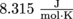3. 9.2 × 1024 molecules

Time for the other version of the ideal gas law. Here we solve PV = NkBT for N, using a temperature of 315 K, a volume of 0.2 m3, and a pressure of 2 × 105 Pa. Also, don't forget Boltzmann's Constant,.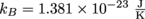4. 0.006 Pa

Assuming that the balloon doesn't pop under these conditions would make this calculation a moot point, so this is a PV = NkBT question; we use this equation since have the number of molecules rather than the number of moles. Except…we don't have the volume. We need to find that first, by using the the 12 cm diameter in meters, which is 0.12 m because there are 2.54 cm in an inch.

The volume of a sphere is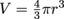, and the radius of the balloon is half of the diameter, or 0.06 m. Therefore, the volume is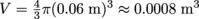.

Next we solve PV = N kBT for pressure:

P(0.0008 m3) = (10 × 1014) (1.38 × 10-23) (350 K)

P = 0.006 Pa

5. 1.5 T1

We know that when Hera decreases the volume of a gas in a closed system (such as a balloon), its internal energy increases, as well as its temperature. But we can do better than that—we can calculate the temperature increase.

Because the total amount of gas doesn't change, we can use this form of the ideal gas law: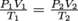. We know that the pressure is tripled, so P2 = 3P1, and that the volume is reduced two-fold, so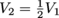. We can now plug these values into our equation and solve for T2.For those who don't like working purely with variables like this, we could also just solve this with made up numbers. We could select arbitrary values for P1 and V1 (we're partial to 2 and 11.726509 ourselves). The actual numbers don't matter, because anything we choose will use a P2 three times as big as P1 and V2 that's only half of V1, all leading to T2 = 1.5T1.

### Kinetic Theory of Gases Solutions

1. 2990.7 J

We need to solve for the total internal energy, U. We are given N = NA = 6.02 × 1023 and T = 340 K, so we'll shove those in the equation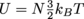.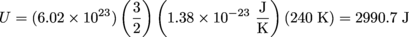2. 4.97 × 10-21 J

Given the number of molecules and the temperature, we need to find the kinetic energy of those molecules. In #1 we used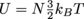to find the U = 2990.7 J. We have a second equation for the internal energy of a system, U = NKavg, so we're set. We know that N = 6.02 × 1023, and we just found U = 2990.7 J, so now we can solve for Kavg, the average kinetic energy of the gas molecules.3.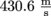In #2 we found the average kinetic energy of a single molecule to be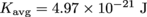. Kinetic energy is also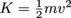, enabling us to solve for velocity.That's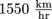or 963.1 mph…pretty speedy! For us that would be speedy, at least. We have less experience with judging molecules.

4. 9.97 × 104 N

Let's break this down into individual steps and look for which equations we need. Why not work our way backwards? What equation tells us something about the force that the gas exerts?

We know that F = PA, so that sounds like the place to start. Uh, end. The box is cubic with sides of 2 cm. We can therefore calculate the area, A, of each wall. We just need to remember that everything has to be in meters.

A = h2 = (0.02 m)2 = 0.0004 m2

Next on the list, we need the pressure, P. So let's make a list of things we do know from questions 1-3 and see what can help us calculate P.

T = 340 K

N = 6.02 × 1023 molecules

Kavg = 4.97 × 10-21 J

U = 2990.7 J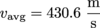m = 5.36 × 10-26 kg

Which equations from the kinetic theory of gases say something about pressure? Oh, here are two. We have all the information necessary for calculating the pressure from either one, a definite bonus.The distance, d, that the gas molecules travel is the height of the box, 0.02 m, so let's go with the first of these equations; we already calculated the area of the side of our box, after all.We can now plug P and A into our force equation to calculate the force that the gas exerts on each wall of the box.

F = PA

F = (2.49 × 108 Pa) (0.0004 m2)

F = 9.97 × 104 N

5. Kavg = 6.17 × 10-21 J

The average kinetic energy of molecules depends only on the temperature. We can use the equation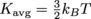to track down T. We gotta convert the temperature into Kelvin as well, though, so it'll play nice with Boltzmann's Constant,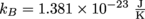.### The First Law of Thermodynamics Solutions

1. Nice. We get to spit out the graphs we saw in the section, and label the area beneath each line as the work done by the system. The isobaric P-V diagram holds constant pressure (horizontal line), the isothermal P-V diagram has a curve, the isovolumetric is a vertical line because of that constant volume (and there's no area under a vertical line) and lastly, the adiabatic line holds energy steady and creates another curve.

(a)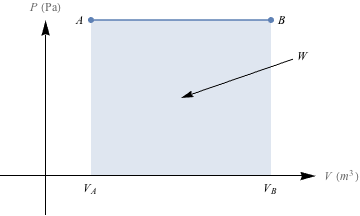(b)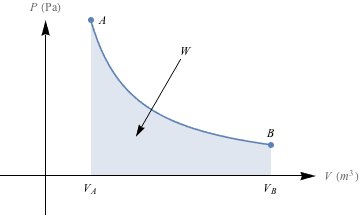(c)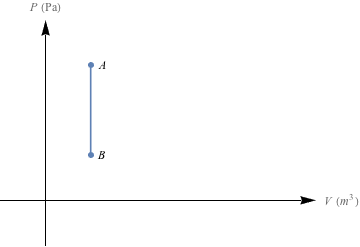(d)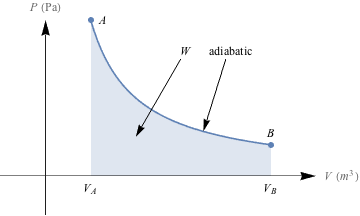2. -108 J

This is an isobaric process (the pressure is constant), and so the work done will be equal to W = -PΔV. Even if we don't remember the equation, we know that the work done by a system is equal to the area under the line on a PV diagram. If we find the area under the line, we'll have found the work, or PΔV.

The change in volume, ΔV, is (6 × 10-3 m3) – (1.5 × 10-3 m3) = 4.5 × 10-3 m3. The pressure, P, is constant at 2.4 × 104 Pa. So, The work is determined by W = -PΔV = -(2.4 × 104 Pa) (4.5 × 10-3 m3) = -108 J.

What about that negative sign, though? The sign is negative because the system is doing work: the initial volume is higher than the final volume.

3. A to B, work done by the system

The amount of work done is equal to the area under the P-V curve. No work is done in the isovolumetric step A to B, so we only compare the area under the isothermal expansion curve A to C to the area under the isobaric expansion curve B to C. The area under B to C is smaller than the area under A to C, so less work is done in the two-step process. Next we calculate the work quantities for each process.

Process B to C is an isobaric process, so the work is W = -PΔV = -P(VfVi). Since Vf > Vi, ΔV has a positive value. W = -PΔV therefore has a negative value, which means that work is done by the system.

Process A to C is an isothermal process, so the work is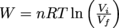. The logarithm is negative when Vi < Vf,, so in the process from A to C, W is negative and work is also done by the system.

4.

-
 W Q ΔU Adiabatic - 0 Isothermal + + 0 Isovolumetric 0 - - Isobaric + + +

5. ΔU = 0 for both cycles, and Cycle 1 does 1800 J of work while Cycle 2 does 900 J of work.

Because each cycle completes a loop and end up where they started, there is no change in energy, U, for either cycle. That sounds like us on Hour 4 of trying to complete our homework for the next day: a lot of work for…not a lot of work.

To calculate the total amount of work done for Cycle 1, notice that work is only done in Steps 2 and 4. Since these are isobaric processes, W = -PΔV.

Wstep2 = − (1 × 105 Pa)[(20 × 10-3 m3) – (2 × 10-3 m3)]

= − (1 × 105 Pa)(18 × 10-3 m3)

= -1800 J

Wstep4 = − (2 × 105 Pa)[(2 × 10-3 m3) – (20 × 10-3 m3)]

= − (2 × 105 Pa)( -18 × 10-3 m3)

= 3600 J

The total amount of work done in Cycle 1, then, is just Wcycle 1 = Wstep2 + Wstep4 = -1800 J + 3600 J = 1800 J.

For Cycle 2, work is done in Steps 2 and 3.

For Step 2, the easiest way to calculate the area under the PV curve is to split the area into a triangle and a rectangle, as shown below.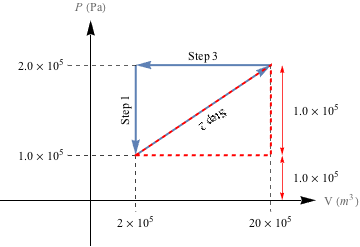Let's calculate the area of the triangle first. The area of a right triangle is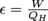The area of the rectangle is W = A = hw.

= (1.0 × 105 Pa) (18 × 10-3 m3)

= 1800 J

The total work done in Step 2 of cycle 2 is the sum of both: Wstep2 = 900 J + 1800 J = 2700 J. Because the gas is expanding during Step 2 as the pressure increases, work is done on the system, so Wstep2 has to be negative: Wstep2 = -2700 J.

Step 3 of cycle 2 is the same isobaric process as step 4 in cycle 1, so the same amount of work, Wstep3 = 3600 J is done. Boom—how's that for saving some brain cells by being clever?

The total amount of work done during cycle 2 is therefore Wcycle2 = Wstep2 + Wstep3 = -2700 J + 3600 J = 900 J.

In both cases work is done on the system, but even though both cycles start and end in the same state, Cycle 1 completes 900 more Joules of work, than Cycle 2.

### The Second Law of Thermodynamics and Heat Engines Solutions

1. ε = 60%

While we don't know why Max would have a heat engine, we do know how to calculate efficiency: from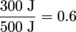This engine is 60% efficient. That's actually highly efficient, as far as engines go. Max's physics teacher should be impressed.

2. Because it has an efficiency of greater than 1.

We're getting 500 J of work for 300 J of heat drawn from the heat reservoir. The second law of thermodynamics forbids such a process. Think about it—where are those extra joules coming from? Knowing our luck, we'd be accidentally drawing power from some extra-dimensional space monster, and that never ends well.

3. QC = 320 J

Here we work backwards from the efficiency formula, sniffing out the energy of the cold resevoir.We're not done yet, since that was the amount of work done by the system, not the energy of the cold reservoir. Since we now know W and QH, we can solve for Qc.

QC = QHW

= 800 J – 480 J

= 320 J

The answer is a chilly 320 joules.

4. ε = 90%Why use words when numbers will do better?

5. W = 283.3 J

We don't have a straightforward equation to go from the efficiency and the cold reservoir's temperature directly to the amount of work done. Instead, we first need to find QH, We can do that with the efficiency equation.Great. Now we're ready to find the work itself.

W = QHQC

= 333.3 J – 50 J

= 283.3 J

Boom; we've got it.

### The Carnot Cycle Solutions

1. εc = 50%

We need the maximum efficiency equation from the Carnot chapter,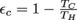. Then we have: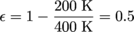'Nuff said.

2. No, because εc = 52%.

The most efficient an engine can be based the Carnot efficiency of the engine.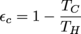Don't go shoving the given numbers into the equation, though. We need to convert the temperatures of the reservoirs to Kelvin, so 300 °C + 273 = 573 K and 4°C + 273 = 277 K. And done. Well, except for actually calculating the maximum efficiency of this engine.The highest efficiency at which this engine can operate is 52%, which is actually quite the achievement for any real-world engine. It's just not up to our exacting (and frankly impossible) standards.

3. TC = 120 K

This is a simple run-n-gun type scenario. In this metaphor, the gun is…wait, give us a second…yeah, it's the efficiency formula we need to use, while the bullets are…the particular values from the question? Look, just roll with it.Okay, that was a terrible metaphor. Sorry about that.

4. 280 K colder

If we first use the efficiency of 50%, we can find the cold reservoir's current temperature.To find the cold reservoir temperature with an efficiency of 90%, we just do the same thing (but with different numbers, obvs).

TC = -TH( εc – 1)

= -700 K (0.9 – 1)

= 70 K

We would have to cool the cold reservoir by 350 K – 70 K = 280 K to get an efficiency of 90%. A cold reservoir of 70 K is getting dangerously close to the impossible-to-hit absolute zero, we'd say.

5. 10%

We've got a lot of plugging away to do into the Carnot efficiency equation.With the original cold reservoir, we calculate the efficiency as 60%.Then with the new temperature, the efficiency increases to 70%.

We want the amount of improvement, which is just the difference between the percentages, 10%.